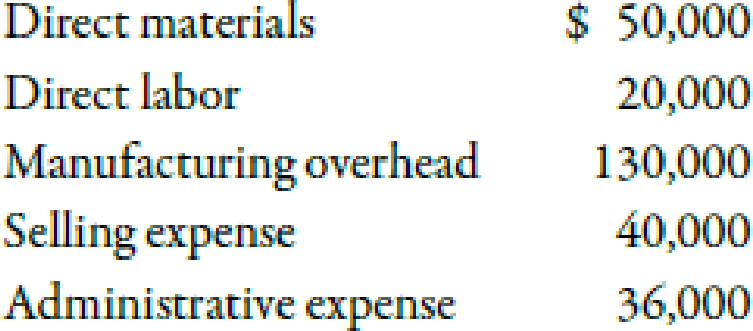Chapter 2, Problem 18MCQ### Managerial Accounting: The Corners...

7th Edition
Maryanne M. Mowen + 2 others
ISBN: 9781337115773

#### Solutions

Chapter
Section### Managerial Accounting: The Corners...

7th Edition
Maryanne M. Mowen + 2 others
ISBN: 9781337115773
Textbook Problem
289 views

# 2-18 Use the following information for Multiple- Choice Questions 2-13 through 2-18:Last year, Barnard Company incurred the following costs:Barnard produced and sold 10,000 units at a price of $31 each.Refer to the information for Barnard Company on the previous page. Operating income is a.$34,000. b. $110,000. c.$234,000. d. $270,000. e.$74,000.

To determine

Identify the amount of operating income.

Explanation

Operating Income:

Operating income can be defined as the difference between gross margin and the total expenses incurred during the normal course of business. All the operating expenses are subtracted from gross margin to calculate operating income.

a.

Use the following formula to calculate the operating income:

Operating income=Sales revenueCost of goods soldPeriod expense

Substitute $310,000 for sales revenue,$200,000 for cost of goods sold and $76,000 for period expense in the above formula. Operating income=$310,000$200,000$76,000=$34,000 The amount of operating income is$34,000. Therefore, this option is correct.

Working Notes:

Calculation of sales revenue:

Sales revenue=Units produced and sold×Selling price per unit=10,000units×$31=$310,000

Hence, the amount of sales revenue is \$310,000

### Still sussing out bartleby?

Check out a sample textbook solution.

See a sample solution

#### The Solution to Your Study Problems

Bartleby provides explanations to thousands of textbook problems written by our experts, many with advanced degrees!

Get Started